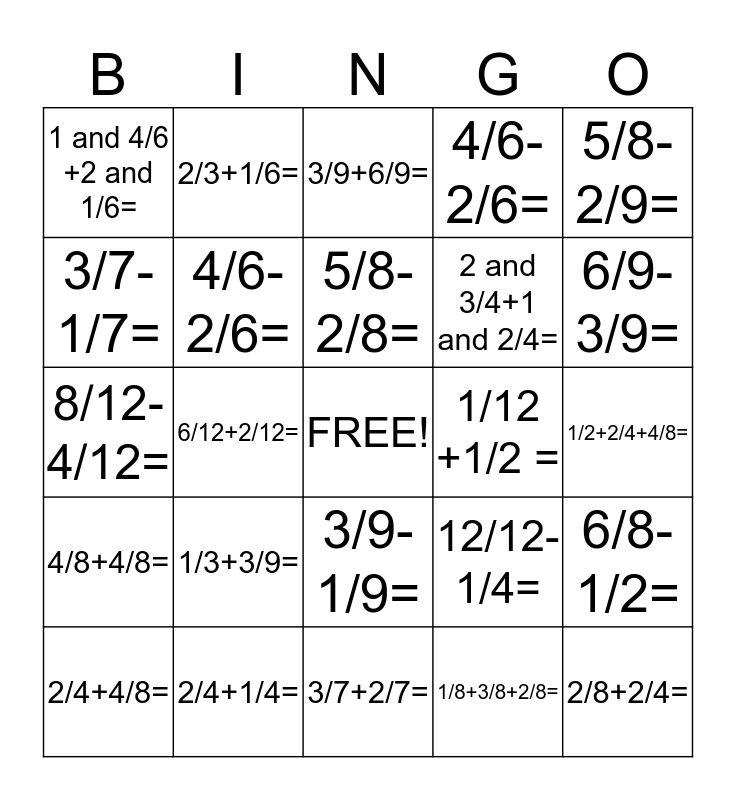# Fraction BingoThis bingo card has a free space and 24 words: 1/3+3/9=, 2/4+1/4=, 5/8-2/8=, 2/4+4/8=, 5/8-2/9=, 1 and 4/6 +2 and 1/6=, 3/7-1/7=, 2/3+1/6=, 1/12 +1/2 =, 3/9-1/9=, 2 and 3/4+1 and 2/4=, 8/12-4/12=, 12/12-1/4=, 6/8-1/2=, 4/6-2/6=, 1/8+3/8+2/8=, 1/2+2/4+4/8=, 3/9+6/9=, 6/9-3/9=, 6/12+2/12=, 2/8+2/4=, 4/8+4/8=, 4/6-2/6= and 3/7+2/7=.

⚠ This card has duplicate items: 4/6-2/6= (2)

## Play Online